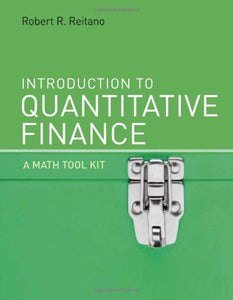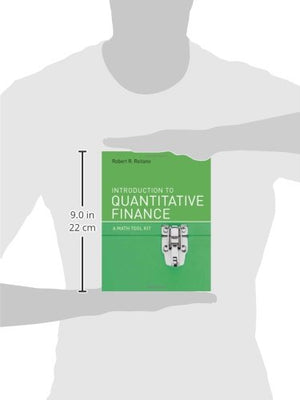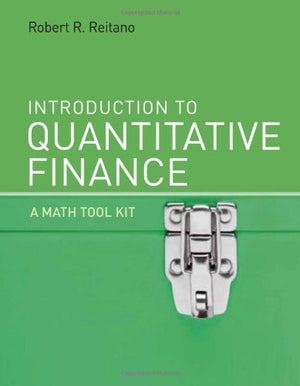Introduction To Quantitative Finance: A Math Tool Kit (Mit Press)

• Publish Date: 2010-01-29
• Binding: Hardcover
• Author: Robert R. Reitano
Regular price \$103.18 Sale price \$80.31

Attention: For textbook, access codes and supplements are not guaranteed with used items.

An introduction to many mathematical topics applicable to quantitative finance that teaches how to think in mathematics rather than simply do mathematics by rote.

This text offers an accessible yet rigorous development of many of the fields of mathematics necessary for success in investment and quantitative finance, covering topics applicable to portfolio theory, investment banking, option pricing, investment, and insurance risk management. The approach emphasizes the mathematical framework provided by each mathematical discipline, and the application of each framework to the solution of finance problems. It emphasizes the thought process and mathematical approach taken to develop each result instead of the memorization of formulas to be applied (or misapplied) automatically. The objective is to provide a deep level of understanding of the relevant mathematical theory and tools that can then be effectively used in practice, to teach students how to think in mathematics rather than simply to do mathematics by rote.

Each chapter covers an area of mathematics such as mathematical logic, Euclidean and other spaces, set theory and topology, sequences and series, probability theory, and calculus, in each case presenting only material that is most important and relevant for quantitative finance. Each chapter includes finance applications that demonstrate the relevance of the material presented. Problem sets are offered on both the mathematical theory and the finance applications sections of each chapter. The logical organization of the book and the judicious selection of topics make the text customizable for a number of courses. The development is self-contained and carefully explained to support disciplined independent study as well. A solutions manual for students provides solutions to the book's Practice Exercises; an instructor's manual offers solutions to the Assignment Exercises as well as other materials.